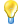CycloBranch
Calculate Molecular Formula

# Calculate Molecular Formula

The tool is opened using the command "Tools -> Calculate Molecular Formula" from the Main Window. Use F1 to open this help.Calculate molecular formula of desferrioxamine E.

## Toolbar

Export Table to CSV (CTRL + E)Export the table of peaks into a csv (comma separated values) file.

Close (Esc)Close window.

Search PubChem... (CTRL + P)Search molecular formulas of compounds in PubChem.

HTML Documentation (F1)Show HTML documentation.

m/z

Enter an experimental m/z value.

Charge

Enter a maximum value of charge. If charge is 2, singly and doubly charged ions are generated and tested. Use negative values if negative ions are selected (e.g., -1).

m/z Error Tolerance

Enter m/z error tolerance value.

Ion Types

Select the types of ions to be calculated. The custom ion types can be defined in the Preferences dialog.

Buttons:

• "Select All" - select all ions in the list.
• "Clear All" - unselect all ions in the list.
• "Reset" - select [M+H]+ ion if defined.

Chemical Elements

Define and select the chemical elements which are used for prediction of compounds. Limit the maximum number of occurrences of an element using a colon (e.g., S:2 means that each formula will contain at the most two atoms of sulfur).

Buttons:

• "Select All" - select all elements in the list.
• "Clear All" - unselect all elements in the list.
• "Add" - add a new item at the end of the list.
• "Remove" - remove selected items from the list.
• "HCON" - clear the list and set the default chemical elements H, C, O, N, S, and P.

Maximum Number of Combined Elements

Enter the maximum number of elements in a formula (M).

Basic Formula Check

Apply Senior's filtering rules. See https://doi.org/10.1186/1471-2105-8-105 for more details.

Advanced filtering rules are applied if molecular formulas of compounds are generated. See the tables 1, 2, and 3 in https://doi.org/10.1186/1471-2105-8-105 for more details.

N/O Ratio Check

Check if the number of nitrogen atoms is less or equal to the number of oxygen atoms (Advanced Formula Check must be enabled).

Calculate

Click on the button to calculate molecular formulas corresponding to the input m/z value.

Cancel

Click on the button to cancel the current calculation process.